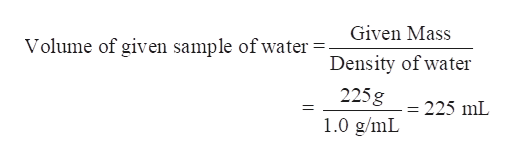# If you had 225 grams of water, what volume would that sample of water occupy? (use your average density you stated above.)

Question
1 views

If you had 225 grams of water, what volume would that sample of water occupy? (use your average density you stated above.)

check_circle

Step 1

We have to determine the volume occupied by the 225 grams of the water sample.

In general, density of water = 1.0 g/mL

(If the average value of density is different, then the solution can be modified as per the value)

Step 2

Density is defined as the mass of the substance divided by its volume.

Step 3

Density of water = 1.0 g/mL

Ma...help_outlineImage TranscriptioncloseGiven Mass Volume of given sample of water = Density of water 225g 225 mL 1.0 g/mL fullscreen

### Want to see the full answer?

See Solution

#### Want to see this answer and more?

Solutions are written by subject experts who are available 24/7. Questions are typically answered within 1 hour.*

See Solution
*Response times may vary by subject and question.
Tagged in

### General Chemistry